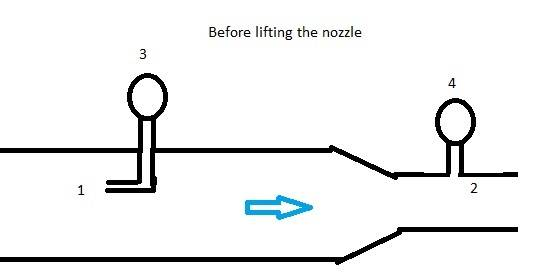# Bernoulli equation / fluid flow / finding height

Polarbear10
Homework Statement:
Bernoulli equation / fluid flow
find height using Bernoulli equation
Relevant Equations:
Bernoulli equation
Qv = V/t = A*V
Hi, everyone! Doing some fluid flow/Bernoulli tasks.

«A hose with a radius of 0,035m is connected to a nozzle, which reduces the radius of the hose to 0,018m (2). The hose carries (qv) 0,0075 m3/sec and the totalpressure (3) in the wide section (1) is 211 kPA. The density of the water is set at 1000 kg/m3.””

• 1: Task one is to find the dynamic pressure in the wide section. I found the velocity to be 1,95 m/s, using V1 = qv/A1. Then calculated the dynamic pressure using Pd = ½ * ρ * v^2 and got 1,89 kPa.
• 2: Second task was to calculate the static pressure. Using Pt = Pd – Ps, and found that PS = 209kPa.
• 3: Then the third task was to find the pressure in the pressure gauge (4). I then calculated the velocity2, using V2=A1*V1/A2, and got 7,37 m/s. I then used Bernouli-equation to calculate the pressure that would be in the gauge (4), and found P2 to be 186 kPa.

4: But the last task: “The nozzle is raised to a higher level. The pressure gauge (3) has a pressure on 241,2 kPa. How high was the nozzle raised”?
I'm struggling to see where I would start. What happens to the pressure in the pressure gauge (4)? Does it change when the nozzle is raised, or will it be the same as in task 3, with P2 being 186 kPa. Also, does the velocity change?

Here is a drawing of the situation, before the nozzle gets lifted.c

•Chestermiller

Homework Helper
Gold Member
The velocity can’ change, as the flowmass must be constant.
You should accont for the additional pressure differential induce by the different height new locations of the gauges.

Polarbear10
The velocity can’ change, as the flowmass must be constant.
You should accont for the additional pressure differential induce by the different height new locations of the gauges.
Hi! Did you mean that the velocity can or can't change since the flowmass must be constant?

But would it be correct to say that P1; is the new pressure on the pressure gauge (3) = 241,2kPa?
And P2 being what I calculated in task 3?

Homework Helper
Gold Member
Hi! Did you mean that the velocity can or can't change since the flowmass must be constant?

But would it be correct to say that P1; is the new pressure on the pressure gauge (3) = 241,2kPa?
And P2 being what I calculated in task 3?
I meant can’t; sorry.
It seems that the pump making the fluid move could compensate for the additional pressure of lifting the hose, by increasing P1 and P3 and keeping the same mass flowing through the pipe.
I seems that we can assume that the original values of P2 and P4 have remained constant.

Polarbear10
I meant can’t; sorry.
It seems that the pump making the fluid move could compensate for the additional pressure of lifting the hose, by increasing P1 and P3 and keeping the same mass flowing through the pipe.
I seems that we can assume that the original values of P2 and P4 have remained constant.
Thanks! That's what I reckon too, so I set up the equation using P1 as 241,2kPa and P2 being 186kPa.
Ended up getting a height (h2) of 3 meters (rounded)..

•Lnewqban